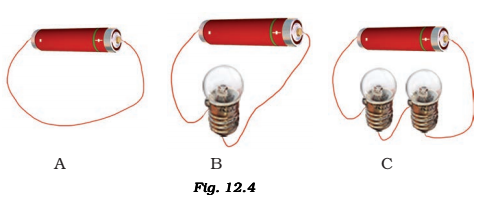In which of the following circuits A, B and C given in the figure, the cell will be used up very rapidly?The cell will be used up rapidly in circuit A as it leads to the loss of total emf of the cell.

• In figure (A) there is no bulb, the circuit is complete but it just connects the negative and positive terminal of the cell. And cell is the source of electricity but in this case we are not using electricity instead current is just flowing through the circuit not using up.
• In figure (B), there is a bulb and the circuit is also complete hence the bulb will glow and will use the electricity and the cell will be used up; but in figure (C) there are 2 bulbs hence consuming electricity at a much faster rate than figure (B) and (A) resulting in a higher rate of consumption of cell and as a result the cell will be used up(4)(3)